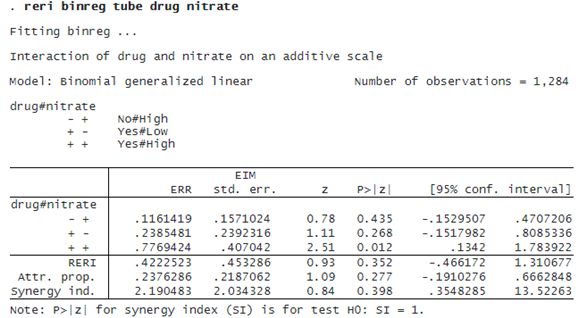RELATIVE EXCESS RISK DUE TO INTERACTION (RERI)

OVERVIEW

The new reri command estimates additive interactions in binomial generalized linear models; logistic, Poisson, and negative binomial regressions; and Cox and other survival models.

Two-way interactions in models that estimate RR are typically expressed as multiplicative interactions. However, for many biological processes, an additive model for the interaction may model the process better than a multiplicative one (see, for example, Andersson et al. ).

For binary exposures A and B, we often want to model the risk of a positive outcome for subjects having both A and B compared with subjects having only A and those having only B. That is, we want to examine the interaction of A and B.

In a multiplicative model, the risk for subjects with A and B is hypothesized to equal the risk for only A times the risk for only B. If the risk for A and B equals the multiplicative risk, we say there is no interaction of A and B. If the risk is greater than the multiplicative risk, we say there is a positive interaction. Multiplicative models are convenient because they are simple to specify, and it is easy to test whether the interaction is greater (or less) than multiplicative.

In an additive model, the risk for subjects with A and B is hypothesized to equal the risk for only A plus the risk for only B. For this hypothesis, we want to look at how much the risk for A and B is greater (or less) than the additive risk.

The RERI statistic is this risk difference formulated using RRs (Rothman, Greenland, and Lash 2008). A RERI statistic of 0 means the risk is additive. A positive RERI statistic means the risk is superadditive, and a negative RERI statistic means the risk is subadditive. The test of statistical significance of the RERI statistic is straightforward, but it is not as simple as the test for the multiplicative model.

Besides the RERI statistic, the reri command reports two other related statistics: AP and SI. AP is the proportion of risk of A and B due to the superadditivity of exposures A and B. SI recasts the RERI statistic as a ratio rather than a difference.

RELATIVE EXCESS RISK DUE TO INTERACTION (RERI) IN ACTION

We illustrate reri with an example that estimates the risk of birth defects from exposures to nitrates. We use a simulated dataset (nitrates.dta) that mimics the results from Brender et al. (2013).

```. webuse nitrates
(Simulated prenatal nitrate intake and birth defects data)
```

Our outcome measure is tube, representing neural tube defects. The exposures are two measures of nitrate intake: drug, with values 0/1, indicating nitrosatable drug exposure; and nitrate, also with values 0/1, representing either low or high daily consumption of nitrates from drinking water.

We want to fit an additive model for the interaction of drug and nitrate. Because this is an observational study, a binomial model of risk is appropriate, and we fit it using binreg:The interactions created by reri represent disjoint groups in the data. The -+ interaction is for drug = No and nitrate = High. The +- and ++ interactions are defined similarly, as shown in the legend. The interaction  is the reference category.

The estimates next to -++-, and ++ are measures of excess relative risk (ERR), defined as RR minus one. ERR greater than 0 means that RR is greater than 1.

If the model is additive, we would have

`  ERR(++) = ERR(-+) + ERR(+-)`

The RERI statistic is the difference between the two sides of this equation:

`  RERI = ERR(++) - ERR(-+) - ERR(+-)`

The estimate of ERR(++) is 0.777, which is greater than ERR(-+) + ERR(+-) = 0.116 + 0.239 = 0.355. So the model is superadditive. The RERI statistic is 0.422, the difference between 0.777 and 0.355.

The AP statistic is the proportion of risk for the ++ interaction that is due to the risk that is above additive. In our example, the estimate of AP is 0.24. The SI statistic is the excess risk expressed as a ratio rather than a difference. Its estimate of 2.19 is greater than 1, which implies a positive interaction or superadditivity.

As well as binreg models, reri can fit logisticpoissonnbregstcoxstintcoxstreg, and stintreg models.